1. /
2. CBSE
3. /
4. Class 12
5. /
6. Chemistry
7. /
8. CBSE Question Paper 2018...

# CBSE Question Paper 2018 class 12 Chemistry### myCBSEguide App

Download the app to get CBSE Sample Papers 2023-24, NCERT Solutions (Revised), Most Important Questions, Previous Year Question Bank, Mock Tests, and Detailed Notes.

CBSE Question Paper 2018 class 12 Chemistry conducted by Central Board of Secondary Education, New Delhi in the month of March 2018. CBSE previous year question papers with solution are available in myCBSEguide mobile app and cbse guide website. The Best CBSE App for students and teachers is myCBSEguide which provides complete study material and practice papers to cbse schools in India and abroad.

CBSE Question Paper 2018 class 12 Chemistry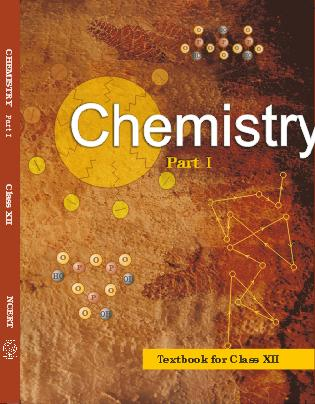## Class 12 Chemistry list of chapters

1. The Solid State
2. Solutions
3. Electrochemistry
4. Chemical Kinetics
5. Surface Chemistry
6. General Principles and Processes of Isolation of Elements
7. The p-Block Elements
8. The d and f Block Elements
9. Coordination Compounds
10. Haloalkanes and Haloarenes
11. Alcohols, Phenols and Ethers
12. Aldehydes, Ketones and Carboxylic Acids
13. Amines
14. Biomolecules
15. Polymers
16. Chemistry in Everyday life

## CBSE Question Paper 2018 class 12 Chemistry

Time allowed: 3 hours
Maximum Marks: 70

General Instructions:

1. All questions are compulsory.
2. Questions number 1 to 5 are very short answer questions and carry 1 mark each.
3. Questions number 6 to 10 are short answer questions and carry 2 marks each.
4. Questions number 11 to 22 are also short answer questions and carry 3 marks each.
5. Question number 23 is a value based question and carries 4 marks.
6. Questions number 24 to 26 are long answer questions and carry 5 marks each.
7. Use log tables, if necessary. Use of calculators is not allowed.

1. Analysis shows that FeO has a non-stoichiometric composition with formula Fe0·95O. Give reason.
Ans.
Shows metal deficiency defect / It is a mixture of Fe2+ and Fe3+/Some Fe2+ ions are replaced by Fe3+ / Some of the ferrous ions get oxidised to ferric ions.
2. CO (g) and H2 (g) react to give different products in the presence of different catalysts. Which ability of the catalyst is shown by these reactions?
Ans.
Selectivity of a catalyst
3. Write the coordination number and oxidation state of Platinum in the complex [Pt(en)2Cl2].
Ans.
Coordination Number = 6, Oxidation State = +2
4. Out of chlorobenzene and benzyl chloride, which one gets easily hydrolysed by aqueous NaOH and why?
Ans.
Benzyl chloride; Due to resonance, stable benzyl carbocation is formed
5. Write the IUPAC name of the following: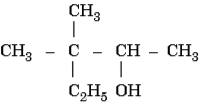Ans.
3,3 – Dimethylpentan-2-ol
6. Calculate the freezing point of a solution containing 60 g of glucose (Molar mass = 180 g mol-1) in 250 g of water.
(Kf of water = 1·86 K kg mol-1)
Ans.= 2.48 K2.48 = 273.15 – Tf
Tf = 270.67 K/270.52 K/-2.48 oC
7. For the reactionthe rate of formation of NO2 (g) is. Calculate the rate of disappearance of N2O5 (g).
Ans.Rate of disappearance of N2O5(Deduct half mark if unit is wrong or not written)
8. Among the hydrides of Group-15 elements, which have the
1. lowest boiling point?
2. maximum basic character?
3. highest bond angle?
4. maximum reducing character?

Ans.

1. PH3
2. NH3
3. NH3
4. BiH3
9. How do you convert the following?
1. Ethanal to Propanone
2. Toluene to Benzoic acid OR

Account for the following:

1. Aromatic carboxylic acids do not undergo Friedel-Crafts reaction.
2. pKa value of 4-nitrobenzoic acid is lower than that of benzoic acid.

Ans.

1.2.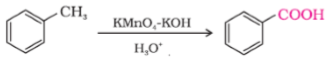OR
1. because the carboxyl group is deactivating and the catalyst aluminium chloride (Lewis acid) gets bonded to the carboxyl group
2. Nitro group is an electron withdrawing group (-I effect) so it stabilises the carboxylate anion and strengthens the acid / Due to the presence of an electron withdrawing Nitro group (-I effect).
10. Complete and balance the following chemical equations:
1.2.Ans.

1.2.11. Give reasons for the following:
1. Measurement of osmotic pressure method is preferred for the determination of molar masses of macromolecules such as proteins and polymers.
2. Aquatic animals are more comfortable in cold water than in warm water.
3. Elevation of boiling point of 1 M KCl solution is nearly double than that of 1 M sugar solution.

Ans.

1. As compared to other colligative properties, its magnitude is large even for very dilute solutions/macromolecules are generally not stable at higher temperatures and polymers have poor solubility/pressure measurement is around the room temperature and the molarity of the solution is used instead of molality.
2. Because oxygen is more soluble in cold water or at low temperature.
3. Due to dissociation of KCl / KCl (aq)K+ + Cl, i is nearly equal to 2
12. An element ‘X’ (At. mass = 40 g mol-1) having f.c.c. structure has unit cell edge length of 400 pm. Calculate the density of ‘X’ and the number of unit cells in 4 g of ‘X’..
Ans.= 4.15 g/cm3
No of unit cells = total no of atoms /413. A first order reaction is 50% completed in 40 minutes at 300 K and in 20 minutes at 320 K. Calculate the activation energy of the reaction. (Given : log 2 = 0·3010, log 4 = 0·6021, R = 8·314 JK-1 mol-1).
Ans. k2 = 0.693/20,
k1 = 0.693/40Ea = 27663.8 J/mol or 27.66 kJ/mol
14. What happens when
1. a freshly prepared precipitate of Fe(OH)3 is shaken with a small amount of FeCl3 solution ?
2. persistent dialysis of a colloidal solution is carried out ?
3. an emulsion is centrifuged?

Ans.

1. Peptisation occurs / Colloidal solution of Fe(OH)3 is formed
2. Coagulation occurs
3. Demulsification or breaks into constituent liquids
15. Write the chemical reactions involved in the process of extraction of Gold. Explain the role of dilute NaCN and Zn in this process.
Ans.
4Au(S) + 8CN(aq) + 2H2O(aq) + O2(g)4[Au(CN)2](aq) + 4OH(aq)
2[Au(CN)2](aq) + Zn(s)2Au(S) + [Zn(CN)4]2- (aq)
NaCN leaches gold/NaCN acts as a leacing agent / complexing agent Zn acts as reducing agent / Zn displaces gold.
16. Give reasons :
1. Eo value for Mn3+/Mn2+ couple is much more positive than that for Fe3+/Fe2+.
2. Iron has higher enthalpy of atomization than that of copper.
3. Sc3+ is colourless in aqueous solution whereas Ti3+ is coloured.

Ans.

1. The comparatively high value for Mn shows that Mn2+(d5) is particularly stable / Much larger third ionisation energy of Mn (where the required change is from d5 to d4)
2. Due to higher number of unpaired electrons.
3. Absence of unpaired d- electron in Sc3+ whereas in Ti3+ there is one unpaired electron or Ti3+ shows d-d transition.
1. Identify the chiral molecule in the following pair: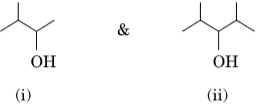2. Write the structure of the product when chlorobenzene is treated with methyl chloride in the presence of sodium metal and dry ether.
3. Write the structure of the alkene formed by dehydrohalogenation of 1-bromo-1-methylcyclohexane with alcoholic KOH.

Ans.

1. (i) /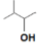2.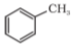3.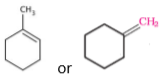17. (A), (B) and (C) are three non-cyclic functional isomers of a carbonyl compound with molecular formula C4H8O. Isomers (A) and (C) give positive Tollens’ test whereas isomer (B) does not give Tollens’ test but gives positive Iodoform test. Isomers (A) and (B) on reduction with Zn(Hg)/conc. HCl give the same product (D).
1. Write the structures of (A), (B), (C) and (D).
2. Out of (A), (B) and (C) isomers, which one is least reactive towards addition of HCN?
Ans.
1. A= CH3CH2CH2CHO
B = CH3COCH2CH3
C= (CH3)2CHCHO
D= CH3CH2CH2CH3
2. B
• Write the structures of the main products in the following reactions :
1.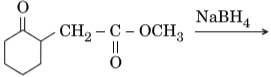2.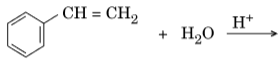3.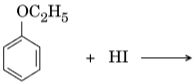Ans.

1.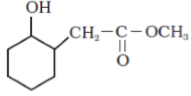2. C6H5CH(OH)CH3
3. C2H5I + C6H5OH (No splitting of marks)
1. Why is bithional added to soap ?
2. What is tincture of iodine? Write its one use.
3. Among the following, which one acts as a food preservative ?
Aspartame, Aspirin, Sodium Benzoate, Paracetamol

Ans.

1. To impart antiseptic properties
2. 2-3% solution of iodine in alcohol – water mixture / iodine dissolved in alcohol, used as an antiseptic/ applied on wounds.
3. Sodium benzoate / Aspartame

These are first 20 questions only. To view and download complete question paper with solution

the best CBSE App from google playstore now or login to our Student Dashboard.

## Chemistry Question Paper 2018 with solution

Download class 12 Chemistry question paper with solution from best CBSE App the myCBSEguide. CBSE class 12 Chemistry question paper 2018 in PDF format with solution will help you to understand the latest question paper pattern and marking scheme of the CBSE board examination. You will get to know the difficulty level of the question paper. CBSE question papers 2018 for class 12 Chemistry have 26 questions with solution.

## Previous Year Question Paper for class 12

CBSE question papers 2018, 2017, 2016, 2015, 2014, 2013, 2012, 2011, 2010, 209, 2008, 2007, 2006, 2005 and so on for all the subjects are available under this download link. Practicing real question paper certainly helps students to get confidence and improve performance in weak areas.

To download CBSE Question Paper 2018 class 12 Accountancy, Chemistry, Physics, History, Political Science, Economics, Geography, Computer Science, Home Science, Accountancy, Business Studies and Home Science; do check myCBSEguide app or website. myCBSEguide provides sample papers with solution, test papers for chapter-wise practice, NCERT solutions, NCERT Exemplar solutions, quick revision notes for ready reference, CBSE guess papers and CBSE important question papers. Sample Paper all are made available through the best app for CBSE students and myCBSEguide website.Test Generator

Create question paper PDF and online tests with your own name & logo in minutes.myCBSEguide

Question Bank, Mock Tests, Exam Papers, NCERT Solutions, Sample Papers, Notes